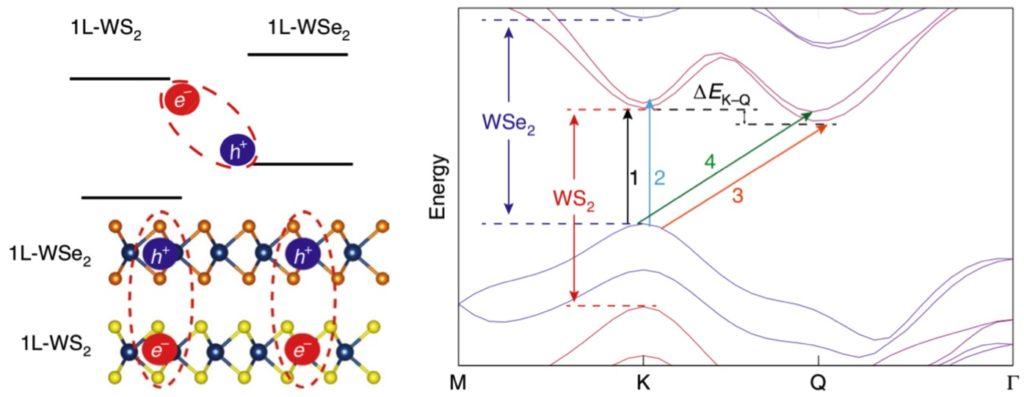# Excitons with a twist: 2D optoelectronics

A recent experimental and theoretical study uncovers the effect of twisting layers in van der Waals heterostructures. Exciton transport in heterobilayers of transition metal dichalcogenides (TMDCs, MXenes) is found to be modulated by the twist angle of the two layers which creates a moiré potential.

Transient absorption microscopy shows longer exciton decay rates at higher temperatures in WS2–WSe2 heterobilayers, even more so when the layers are twisted by 60°. Theoreticians Agnieszka Kuc, Thomas Brumme, and Jens Kunstmann explained the observed exciton dynamics. Band structure calculations on the heterobilayers were performed with spin-orbit coupling DFT calculations at 0° and 60° twist angle under 2D periodic boundary conditions with BAND.

In heterobilayer WS2–WSe2 excitons the hole preferentially resides in the WSe2 valence band, while the electron moves in the WS2 conduction band. In this conduction band, the Q-point is lower in energy than the K-point. Since K-K exciton decay is now thermally activated, a higher temperature leads to higher population of K electrons, resulting in longer decay rates. Since the energy difference ΔEK–Q is lower at a 60° twist angle than at 0°, the Q to K backscattering is more efficient, leading to a longer K-K decay rate.WS2–WSe2 heterobilayers have energetically favorable K-Q interlayer excitons, with the lowest transition between the K valence band maximum and Q conduction band minimum. Direct K-K decay is thus thermally activated. ΔEK–Q and thus the decay rate is twist-angle dependent.

Example input files are available. Note that there is a lattice mismatch of about 4% between the WS2 and WSe2 monolayer (ML) lattices. A fully commensurate lattice would require thousands of atoms in a unit cell, for which spin-orbit coupling DFT calculations are currently not feasible. Instead, symmetric 6-atom systems were used with three lattices: at the WS2-ML relaxed lattice, the WSe2-ML relaxed lattice, and an averaged lattice. The latter strains WS2 and compresses WSe2, which leads to the incorrect band structure.

Twisting is just one of the possibilities to tweak the optoelectronic properties of 2D semiconductors. These insights on how twisting in heterobilayers affects exciton dynamics, guided by density functional calculations will help to further develop quantum photonics devices.

We will further develop methods to study the optoelectronic properties in 2D semiconductors in an upcoming EU project.